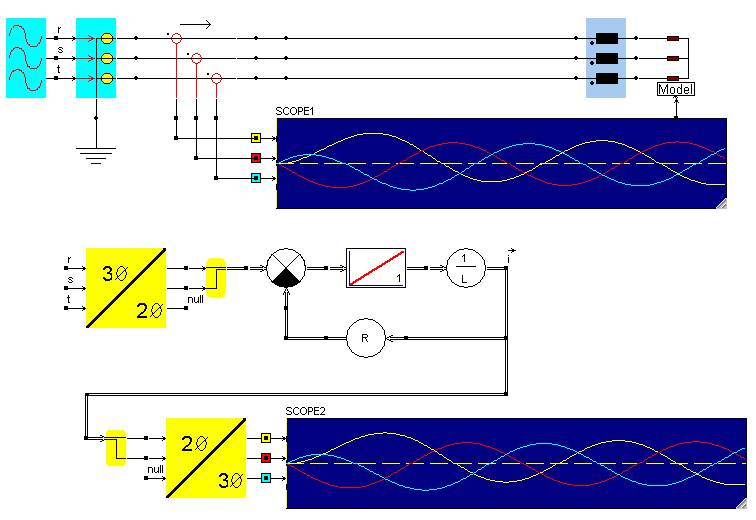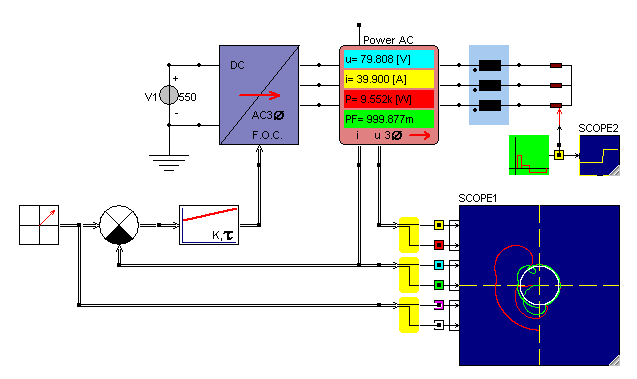• Introduction
• What is in this manual
• What is Caspoc
• User interface
• Introduction
• Starting
• Simulation
• Editing
• Viewing and printing
• Getting Started
• Basic editing
• Simulation in the time domain
• Basic User Interface Topics
• Editing
• Simulation
• Viewing
• Library
• Reports
• Project management
• Circuit and Block Diagram Components
• Introduction
• Cscript and user defined functions
• Component parameters
• Modeling Topics
• Introduction
• Power Electronics
• Semiconductors
• Electrical Machines
• Electrical drives
• Power Systems
• Mechanical Systems
• Thermal Systems
• Magnetic Circuits
• Green Energy
• Coupling to FEM
• Experimenter
• Analog hardware description language
• Embedded C code Export
• Coupling to Spice
• Small Signal Analysis
• Matlab coupling
• Tips and tricks
• Appendices

## Mixing the space vector format with the electric circuit.

Equivalent space vector model
Using the components from the Advanced Electrical Drives library, equivalent systems can be constructed to model three phase electric circuits. The example below shows a three phase grid with inductive-resistive load. The circuit is also modeled using space vector components.
The grid voltages are at the nodes r, s and t and are transformed into ab for the space vector component model.PI control of voltage source inverter
The simulation below shows an ideal three phase inverter with PI control. The currents in the load circuitry are measured and transformed into a two phase system. The PI controller is modeled using the space vector components.